Lesson Plan

## Using Benchmarks to Compare Fractions

### Objectives

In this unit, students are introduced to the concept of fractions and how they can be compared. (Mastery is not expected until grade 5.) Students will:

• compare fractions by using concrete models.
• compare fractions by using benchmarks.
• order fractions by using concrete models, benchmarks, and parallel number lines.
• explore fair-sharing situations with unit fractions.

#### Essential Questions

How are relationships represented mathematically?
How can mathematics support effective communication?
How is mathematics used to quantify, compare, represent, and model numbers?
What does it mean to estimate or analyze numerical quantities?
What makes a tool and/or strategy appropriate for a given task?
• How is mathematics used to quantify, compare, represent, and model numbers?
• How are relationships represented mathematically?
• What does it mean to estimate or analyze numerical quantities?
• What makes a tool and/or strategy appropriate for a given task?

### Vocabulary

• Denominator: In a fraction, the number or quantity below the fraction bar. Tells the number of equal parts into which a whole is divided.
• Fraction: Notation used to represent part of a whole or part of a group by telling the number of equal parts in the whole (denominator), and the number of those parts being described (numerator).
• Inequality: A mathematical sentence that contains a symbol (<. >, ≤ , ≥, or ≠) in which the terms on either side of the symbol are unequal.
• Mixed Number: The sum of a whole number and a fraction.
• Numerator: In a fraction, the number or quantity above the fraction bar. Tells the number of parts of a whole being described.
• Unit Fraction: A fraction with a numerator of 1.

90–120 minutes

### Prerequisite Skills

Prerequisite Skills haven't been entered into the lesson plan.

### Related Materials & Resources

The possible inclusion of commercial websites below is not an implied endorsement of their products, which are not free, and are not required for this lesson plan.

### Formative Assessment

• View
• Your observations during lesson activities and classroom discussion will help in evaluating student skill levels in comparing fractions.
• Have students complete the Lesson 1 Assessment.
• Use the comparisons recorded during the Small Group Extension activity to further assess student progress.

### Suggested Instructional Supports

• View
Scaffolding, Active Engagement, Modeling, Formative Assessment W: Students review the concept of a fraction and terminology of a fraction. H: Students use manipulatives to compare fractions and to determine which fraction is greater. E: Students examine benchmark fractions and 0,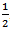, and 1. R: Students review what they learned during the benchmark fraction activity and discuss what patterns they found to help with fraction comparisons. E: Student understanding is evaluated through discussions and questions as well as by giving the Lesson 1 Assessment. T: The lesson may be tailored to meet the differing needs of students. Students who may need additional practice would benefit from comparing fractions using the same denominator. For a greater challenge, students may extend the idea of comparing fractions by using improper fractions. O: Students use different types of fraction manipulatives to help them compare and order fractions.

### Instructional Procedures

• View

Note: Blank templates for fraction strips and fraction circles are available if needed (M-4-3-1_Fraction Circle Template.doc and M-4-3-1_Fraction Strip Template.doc).

Say to the class, “Let’s review some of the things we know about fractions. A fraction represents part of a whole unit or part of a group of objects. Fractional parts of a whole unit must be equal in size.”

Draw the following pictures on the board.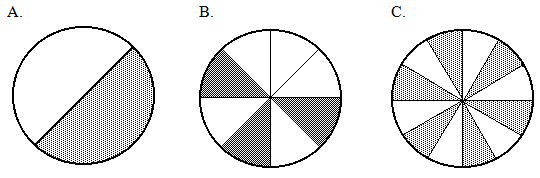Write the following on the board: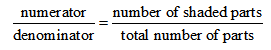Have a student name the fraction for the shaded part of each circle: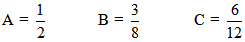Ask “Which is more?” questions, starting with the samples on the board. Point out that figures A and C show two representations of one-half and are equal, although both the numerator and denominator in figure C are larger than those in figure A. Also have students explain in their own words how they know that figure B represents a fraction that is less than the others and what it would take to make it equal or greater than one-half. Encourage students to demonstrate their thinking using fraction circles, chips, 1-inch color tiles, pictures, or number lines.

• “Which is more,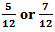of a dozen eggs?”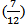• “Which is more,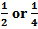of a pie?”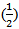• “Which is more,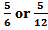of an energy bar?”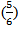• “Which is more,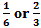of a pizza?”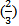Students’ responses will provide a quick check of their skills in comparing and ordering fractions. Introduce the term benchmark fractions to students. Explain that benchmark fractions are commonly used fractions. Tell them, “We will be comparing fractions to 0,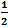, and 1.”

Have students fold a sheet of paper to create a three-column benchmark chart and record their answers in the chart.

 Fractions Nearest to 0 Fractions Nearest to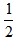Fractions Nearest to 1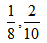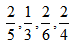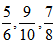On the board or overhead transparency, write the following fractions: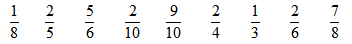Have students analyze each fraction in relation to 0,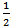, and 1. Provide parallel number lines (M-4-3-1_Parallel Number Line Sheet.doc) or fraction circles for students to use. Ask,

• “Which fractions are closest to 0?
• Which fractions are closest to?
• Which fractions are closest to 1?
• Do you see any patterns among the fractions in each column?”

Help students discover and describe the following patterns between the fractions in each column:

• Fractions closest to 0 have numerators that are very small in comparison to their denominators.
• Fractions closest tohave denominators that are about twice as great as their numerators, or the numerators are half as great as their denominators.
• Fractions closest to 1 have numerators and denominators that are about equal.

Write the following groups of fractions on the board: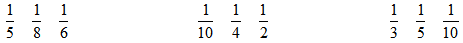Have students discuss how to order each group of fractions from least to greatest. Have students use the Parallel Number Line worksheet.

When ordering unit fractions from least to greatest, the fraction with the largest denominator has the smallest value.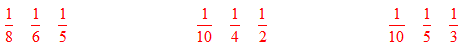Note: Be sure students understand the above pattern only applies when the numerators are all 1.

Say to the class, “Now that you can order fractions, we are going to compare fractions using the symbols <, >, or =.” Write and draw the following on the board: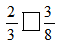Be sure to point out that the fraction strips are equal in length.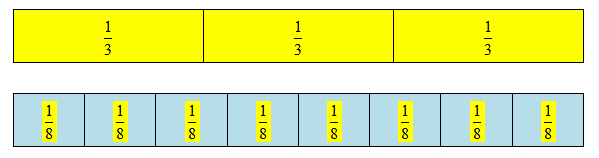Ask for a student volunteer to circle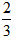and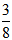on the fraction strips.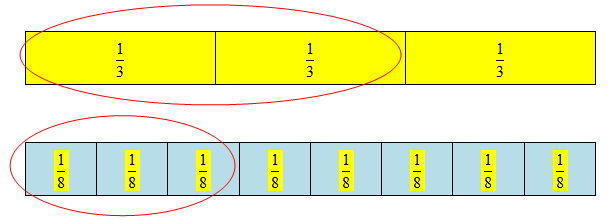Ask, “Which fraction is greater?” Have another student place the appropriate symbol in the box.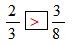For additional practice, write the following problems on the board (without the symbols filled in).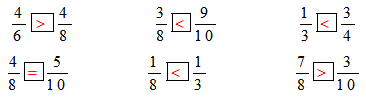Give students a few minutes to do the practice problems. Call on students to supply the answers and discuss any misunderstandings.

“Now that we know more about comparing fractions we will look at this problem.” Present a “fair-share” problem. Students should work with a partner.

Jenny, Marcus, and Renee bought two 1-foot-long submarine sandwiches. They want to share the sandwiches equally. How much should each of the three friends get? Draw a picture of the context of the problem on chart paper.

Ask students to talk to their partners for a few minutes about how to divide the two sandwiches equally among three people.

• “How much does each friend get?” (of each sandwich or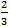of the total sandwiches)
• “How is it possible for three people to share two sandwiches?” (They divided each sandwich by thirds to make 6 parts and took 2 parts each.)

Encourage students to use any materials they find useful to compare the fractions (paper strips to fold, linking cubes) and to make drawings to help them. It is important that students construct a way to show the problem. Do not provide materials with the fractions already labeled. Making and drawing the fractional pieces encourages students to think about the meaning of the fractions.

For additional practice, have students complete the Fair Share Problems worksheet (M-4-3-1_Fair Share Problems and KEY.doc).

When students have completed all activities, discuss the results and clear up any confusion. Then have each of them complete the Lesson 1 Assessment (M-4-3-1_Lesson 1 Assessment and KEY.doc).

Extension:

• Routine: Create a set of fraction cards and randomly select two cards. Have students select the greater or lesser fraction of the pair. Repeat the activity for daily review or to remind students how to compare fractions throughout the school year.
• Small Group: Separate the class into groups of two (pairs). Give each student a number cube. Have students write a fraction with a denominator of 7 and a blank numerator. Have students roll the number cube and use the number rolled as the numerator of their fraction. Encourage students to compare and record each pair of fractions. Repeat this activity using other denominators greater than 6.
• Expansion:
• Have students determine which benchmark fractions, 0,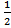, or 1, the given fractions are nearer. Include fractions that are equally between the two benchmarks for students to discuss (for example,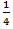and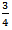are equidistant from). A resource worksheet is available to help students organize work (M-4-3-1_Expansion Resource Sheet.doc).
• To extend the idea, list several improper fractions and have students determine the wholes or halves each is nearer to. For example,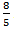is between 1 and 2 but nearer to 2, or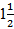. (For additional examples, use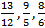).

### Related Instructional Videos

Note: Video playback may not work on all devices.
Instructional videos haven't been assigned to the lesson plan.
Final 05/16/2013